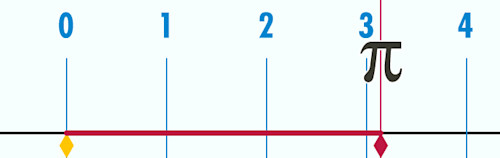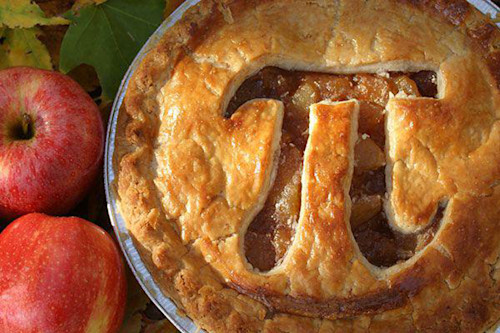Your weather when it really mattersTM

Circle the date! It's Pi Day today!

Thursday, March 14th 2019, 4:04 pm - Happy Pi Day, the annual celebration of this incredible, mysterious and irrational mathematical constant.

Okay, so π (or Pi) a mathematical constant.

It's equal to 3.14, or 3.1415926 or 3.1415926535897932384626433832795 - depending on what kind of calculator you use, although it goes on for much longer (see below).

We use it in calculations in geometry and trigonometry, mainly having to do with circles and ellipses and so forth, and by extension, it shows up in the sciences - like physics, astronomy and meteorology, just to name a few examples.

BUT WHAT IS π, REALLY?

Simply enough, π is the ratio of a circle's circumference to its diameter.

Draw a circle 1 metre across and its circumference will be π metres. A circle 1 centimetre across will be π centimetres around.The circumference of a circle being traced out π in units of its diameter. Credit: Wikimedia Commons

OK, BUT WHAT MAKES π SO SPECIAL?

π is an irrational number - one that never repeats and never ends. As such, to the best of our considerable knowledge of mathematics and the universe, we'll probably never know its exact value.

As the above circle unrolls, and it's compared to a ruler laid on the ground, the end of the circle will not fall exactly on one of the marks on the ruler. In fact, no matter how close together those marks are, or how small of a space they measure, or how closesly we zoom in on the ruler, the end of the circle will never fall exactly on the lines, ever.

As of 2019, π has been calculated to 31,415,926,535,897 decimal places, which was accomplished by Emma Haraku Iwao, by using Google Cloud technology.Even with that incredible feat of computation, we still haven't found the end of π, nor have we found any repeating pattern in the numbers, and it's very likely that we never will, no matter how much knowledge we accumulate or how powerful our computers become! That's why asking a computer or robot to compute the last digit of was such a good trick to use in science fiction stories. If the computer or robot didn't overload in the process, it at least bought you some time to escape.

Perhaps if we were able to look at our universe from the outside, we'd be able to see π resolve down to a simple, rational number. From here, inside the universe, this mathematical constant holds as an enigma of the cosmos.

Fortunately, the cosmos is forgiving enough that we don't need to know π to the last digit in order to produce useful results.

CLICK BELOW TO WATCH: The fine people at YouTube's Numberphile (who appropriately use π as their user icon) show how to calculate π, using pies.

π IN WEATHER?

How often do we see π show up in meteorology? More often than you might think!

While there aren't too many exact circles in weather, whenever you have rotation - in convective circulations, turbulence, and in storms - supercells (and the tornadoes they produce), extra-tropical cyclones, and tropical storms and hurricanes - π plays a strong role in that motion!

Take the recent example of the 'Fibonacci Spiral' pattern produced by the storm passing over the United States and Canada this week!

The "Golden Spiral" overlaid onto the storm pattern is one where the radius of the spiral increases in such a way that it matches a Fibonacci number sequence. The sizes of the boxes that the spiral curves through are 1x1, 1x1, 2x2, 3x3, 5x5, 8x8, 13x13 and 21x21 (and it would continue with 34x34, 55x55, 89x89, 144x144, etc).

The radius at any point along the curve, though, can be found with the equation below,where phi (ψ) is the 'golden ratio', which is an irrational number, itself = 1.6180339887..., theta (θ) is the angle from the origin, in radians, and we can see π figuring prominently in the exponent of the equation.

Frankly, we just can't get away from π in weather!

SO, HOW DOES ONE CELEBRATE A MATHEMATICAL CONSTANT?Pi pie, courtesy koka_sexton/Flickr

Conveniently, the name of this particular mathematical constant happens to be a homonym for a very tasty dessert treat - pie!

Apple pie, cherry pie, blueberry pie, strawberry-rhubarb pie ... even pizza pie.

As long as it's round, it will do just fine.

OTHER SPECIAL CONSTANT DAYS?

Do we celebrate any other scientific or mathematical constants? Indeed, we do!

There's Pi Approximation day, on July 22, since 22/7 is a fraction used to approximate π (it comes out as 3.14285714...)

e-day, either on January 27 or February 7 - depending on whether you write your dates day first or month first - celebrates Euler's Constant, e = 2.7182818 (remember your 'natural logarithm', ln?).

For the chemists, there's Mole day, on October 23 (specifically between 6:02 am and 6:02 pm), since one Mole of a substance contains 6.02x1023 atoms or molecules of that substance.

Then there's Tau Day, on June 28, which celebrates τ, a constant that is equal to 2π. Or, π is 1/2τ, depending on which side of the rivalry you're on ... and yes there's a rivalry there!

Why? Because a circle is defined as "the set of all points in a plane that are at a given distance from a given point", or in other words, a circle is defined by its radius, not its diameter. So, the equation for circumference is really C = 2πr = τr. So, if you want to make the argument, τ technically makes a better mathematical constant.

Unfortunately, while τ may be better mathematically, it does not have a tasty food associated with it.

For more on π and to try out some cool uses of π, check out NASA's Pi in the Sky Challenge!

Sources: NASA JPL | C Net | Tau Day

Default saved
Close